# SSAT Middle Level Math : How to find the points on a coordinate plane

## Example Questions

### Example Question #6 : Graphing Points

Which of the following points would be located in Quadrant III?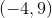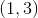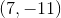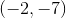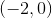Explanation:

By definition, a point on the coordinate plane that is in Quadrant III must have both a negativecoordinate and a negativecoordinate. The only answer choice that satisfies both of these conditions is.

### Example Question #1 : How To Find The Points On A Coordinate Plane

In which quadrant or on what axis will you find the point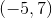?

The-axis

Explanation:

The pointhas a negativecoordinate and a positivecoordinate. By definition, any point on a coordinate plane with these characteristics is located in Quadrant II.

### Example Question #8 : Graphing Points

In which quadrant or on which axis will you find the point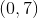?

The-axis

The-axis

The-axis

Explanation:

The pointdoes not move in any direction on the-axis, but does moveunits up on the-axis. Therefore, the point is located only on the-axis.

### Example Question #10 : Graphing Points

Which of the following points is in Quadrant II on the coordinate plane?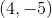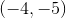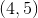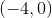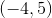Explanation:

All points in Quadrant II have negative-coordinates and positive-coordinates. The only answer that fulfills these criteria is### Example Question #2 : How To Find The Points On A Coordinate Plane

In which quadrant or on which axis will we find the point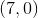?

The-axis

The-axis

The-axis

Explanation:

By definition, any point in the coordinate plane that has a non-zero value-coordinate and a zero-value-coordinate is on the-axis.

### Example Question #1 : How To Find The Points On A Coordinate Plane

In which quadrant or on which axis of the coordinate plane will you find the point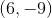?

The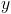-axis

Explanation:

A point with a positive-coordinate and a negative-coordinate can be found in Quadrant IV.

### Example Question #4 : How To Find The Points On A Coordinate Plane

A line segment on the coordinate plane has its endpoints at the points with coordinates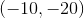and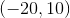. Give the coordinates of the midpoint of the segment.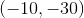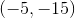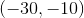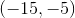Explanation:

The-coordinate of the midpoint can be found by dividing the sum of the-coordinates of the endpoints by 2: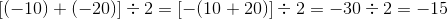The-coordinate of the midpoint is found similarly: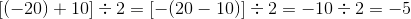The coordinates of the midpoint are.

### Example Question #5 : How To Find The Points On A Coordinate Plane

A line segment on the coordinate plane has its endpoints at the points with coordinates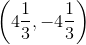and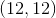. Give the coordinates of the midpoint of the segment.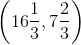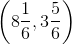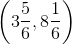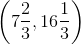Explanation:

The-coordinate of the midpoint is half the sum of the-coordinates of the endpoints: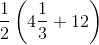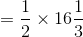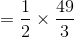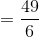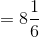The-coordinate of the midpoint is found similarly: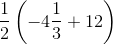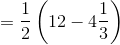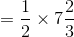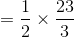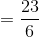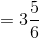The midpoint has coordinates.

### Example Question #9 : Graphing Points

In which quadrant or on which axis will you find the point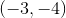?

The-axis

By definition, a point with a negative-coordinate and a negative-coordinate lies in Quadrant III on the coordinate plane.